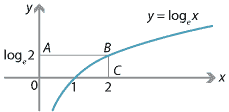### Definite integrals

We illustrate the use of inverses in determining definite integrals through an example.

#### Example

Find

$\int_1^2 \log_e x \;dx.$

#### SolutionDetailed description

We note that $$e^x$$ and $$\log_e x$$ are inverses of each other. From the diagram, we have

\begin{align*} \int_1^2 \log_e x \;dx &= \text{Area of rectangle }OABC - \int_0^{\log_e 2} e^y \,dy \\ &= 2\log_e 2 - \bigl[ e^y \bigr]_0^{\log_e 2} \\ &= 2\log_e 2 - 1. \end{align*}

It is clear that this technique can be used profitably in many similar situations.

Next page - Links forward - Transformations of the plane